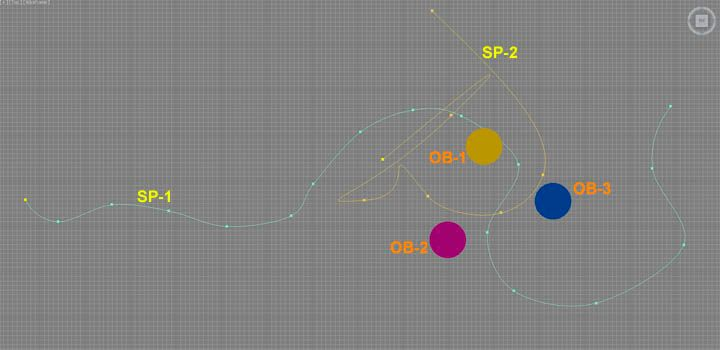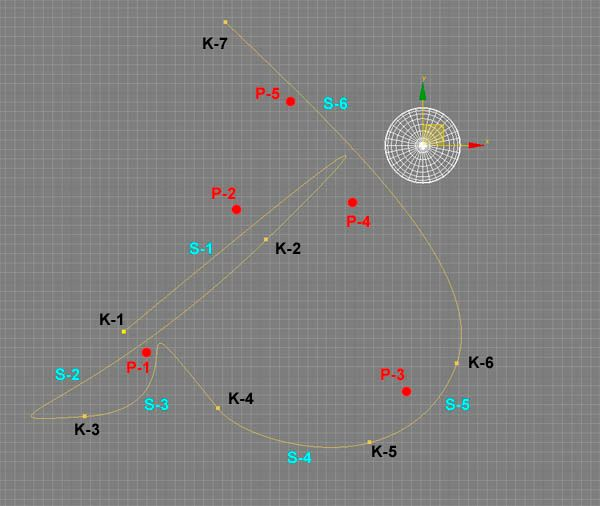# (SDK) what is equivalent to mxs nearestpathparam?

#21

after 4 hours fighting and researching i’ve improved nearestPathParam function and made it ~8 times faster for 1000 steps, ~20 times faster for 10000 steps, and ~200 times faster for 100000 stepsthere is what i started with http://www.cyberforum.ru/geometry/thread1425152.html

but this algorithm works for only quadratic Bezier curves, and it doesn’t work for cubic curves. But!!! any cubic curve can be approximated by several quadratic curves. there is a theory how to break cubic bezier on quadratics. it’s what i actually do.

#22

I can’t follow the code of the link you posted. To hard for me.
But I can’t see where it is limited to second degree. In fact, I see the initial formula of third degree:
B (t) = (1-t)^3 * P1 + 3t * (1-t)^2 * P2 + 3t^2 * (1-t) * P3 + t^3 * P4

What I’ve done is simplify this formula to:
H(t) = cc + cc*t + cc*t^2 + cc*t^3 , thus derivatives are:
H’(t) = cc + 2 *cct + 3cc*t^2
H’’(t) = 2 cc + 6cc*t

The function to find its roots is the dot product F(t) = H(t).H’(t) = 0 (5th degree).
Then you use Newton-Raphson: t_n+1 = t_n - F(t_n) / F’(t_n)

We know that the derivative of a dot product is:
(F(t).G(t))’ = F(t).G’(t) + F’(t).G(t)
So
(H(t).H’(t))’ = H(t).H’’(t) + H’(t).H’(t) = H(t).H’’(t) + lengthsquared(H’(t)).

Returning to Newton-Raphson:
t_n+1 = t_n - (H(t_n).H’(t_n)) / (H(t_n).H’’(t_n) + lengthsquared(H’(t_n)))

I think that this saves a lot of calculations.

The big problem with Max is that Point3 and all its vector operations (dot product for example, or length) are float and not double. So its nearly impossible to approach to a value of ‘t’ with more than 3 exact decimals. For a spline of 1km (1000m), that means you approach to the unit (first decimal at most). That is not very much in most cases.

Using double float calculations (as I have done with Vector3D is c# from “System.Windows.Media.Media3D.Vector3D”) you win 1 decimal (not more because input data Point3 are still float and the ‘t’ param for pathInterp3D too).

#23

to make ‘step-iteration’ faster it uses The bisection method, which is true for ‘convex’ curves (quadratics for sure)

#24

Not bad… and better than mine I think.

These are my results for next cases, with the two bezier splines of the image below and a sphere of 20.000 vertex in different positions:
Comparison between my code in C# with just 7 subsegments, the default MaxScript nearestPathParam and the MaxScript nearestPathParm with steps = (lengh of spline * 10) to reach the same accuracy than the C# code (not the same, but enougth).Spline SP-2 converted to polyline - Sphere in any position:
C#: 0,048s - Default MXC: 4,662s (97 times faster) - MXC steps = lengthx10: 22,027s (460 times faster)

Spline SP-1 converted to polyline - Sphere in any position:
C#: 0,082s - Default MXC: 8,662s (105 times faster) - MXC steps = lengthx10: 42,731s (520 times faster)

Spline SP-1 (bezier) - Sphere in any position:
C#: 1,087s - Default MXC: 9.822s (9 times faster) - MXC steps = lengthx10: 48,513s (45 times faster)

Spline SP-2 (bezier) - Sphere in position 2 or 3 (near few segments):
C#: 0.717s - Default MXC: 6,742s (9,5 times faster) - MXC steps = lengthx10: 33,086s (46 times faster)

Spline SP-2 (bezier) - Sphere in position 1 (near several segments):
C#: 1,175s - Default MXC: 6,746s (6 times faster) - MXC steps = lengthx10: 33,115s (28 times faster)

In cases 3 and 4, I reach the same accuracy with only 5 segments instead of 7, obtaining of 30% more speed.
I haven’t never needed more than 11 subsegments to reach the maximum accuracy.

Edit: SP-1 length = 2341 units, SP-2 length = 1767 units

#25

it sounds like you want better accuracy working with splines of ‘kilometers’ long. but it doesn’t matter sometimes how accurate you calculate Length or DotProduct because input and output data is not accurate enough anyway

#26

could you post a sample scene (max 2014 or less) to make a compare test of our methods (accuracy and performance)?

#27

Yes, of course.
I’ve have sent you a pm.

#28

At last, I think I have something consistent to offer!!

It’s been really hard. But now it seems that everything works.
The algorithms failed in the areas where there are very close possible solutions (in the image below, if you check for a very dense object located in the P-1 area for example, or in the maximum of S-1 and near S-6.In fact, the native Maxscript function fails too in this cases sometimes (if you give the default steps value), giving a “nearpathparam” that is in other segment than the good solution is.
Now, I solved it (at last!)

So, what we have here is:

• A function to search for the nearestPathParam for a set of nodes at a time, faster and more accurate than the native function.
• The use is explained in the attached mxs script and in the C# code.
• For bezier curves, the speed is increased by 10 to 25 in ‘safe mode’ and by 65-100 in ‘screening mode’ comparing with default steps of the native function. For more steps in native function, times are far better.
• For polygons, the speed is far better too (200 to 500 times).
• The accuracy is allways better than 1E-05 for the pathParam.

Use it if you want to. It’s free! Let me know please any problems found.
The attached ZIP contains the maxscript file, the native code in C# and a compiled dll (that I hope works in computers different than mine. I’m an absolute rookie in C#)

Note: speed tests made with a 20k vertex sphere.

#29

Sorry. Deleted. Still doesn’t work in some cases. :banghead:

It seems that consider straight segments based just in comparing distance between knots and segment length is not a good idea… The pathParam of “nearly straight segments” doesn’t have an homogeneous distribution.

#30

Well… Here’s the final version, with some improvements:

• Speeded up by 4 to 6 thanks to Parallel processing (now it’s really very fast).
• Accepts defaults values for spline number and subsegments
• Works with arrays of nodes and arrays of Point3 (two different function names)
• Solved bug with straight segments

Use with MaxScript:

``````(

-------------------------------------------------------------------------
-- USE WITH ARRAY OF GEOMETRY NODES
-------------------------------------------------------------------------
theObjects = selection as array						--	Array with the nodes to search for nearestPathParam of their verts
theHandles = for o in theObjects collect o.inode.handle		--	Get the nodes handles

thePath = \$Line001								-- 	The shape node containing the spline
thePathHandle = thePath.inode.handle				--	Get the shape handle
numSpline = 0																-- 	Number of the spline in the shape (zero based) [default to 0]

subSegments = 1															--	Number of subsegments for calculation [default to 3]. See note below:

--	subSegment = 0: search in every segment of the spline, without segment screening. Gives the best solution in 99% of the cases. (slow)
-- 	             		-n (negative values): search in every segment with n+1 subdivisions. Only needed in very special cases (not need over -2). (slower)
-- 	             		n (positive odd number): prior screening of segments with n subdivisions and n steps per subdivision. (fast)
-- 	             		n (positive even number): prior screening of segments with (n-1) subdivisions and (n+1) steps per subdivision. (fast)
-- 	            		value 1 is the fastest one. Gives the best solution in 75% of the cases. Value of 3 gives solution in 99% of cases. Not need of values over 9.
--				For linear splines (polygons), this value isn't important for time and calculation.
--				[default to 3]

cc = (dotnetclass "Proin3D.SuperNearestPathParameterMain")
c = cc.SuperNearestPathParameterNodes   theHandles   thePathHandle   subSegments   numSpline
--	c = cc.SuperNearestPathParameterNodes   theHandles   thePathHandle   subSegments   	-- Default value for numSpline = 0
--	c = cc.SuperNearestPathParameterNodes   theHandles   thePathHandle      					-- Default value for numSegments = 3

-- 	c variable will contain an array of arrays (one per input node). Each of these arrays contains the nearestPathParam for each vertex of the node

-------------------------------------------------------------------------
-------------------------------------------------------------------------

-------------------------------------------------------------------------
-- USE WITH ARRAY OF POINT3
-------------------------------------------------------------------------
--	Point3List = #([x1,y1,z1], [x2,y2,z2],...)   -- array of Point3
--	List_Point3D = for v in Point3List collect (#(v.x, v.y, v.z))   -- Convert Point3 array to float array

List_Point3D = for v in theObjects.verts collect (#(v.pos.x, v.pos.y, v.pos.z))

c = cc.SuperNearestPathParameterList3D   List_Point3D   thePathHandle   subSegments   numSpline
--	c = cc.SuperNearestPathParameterList3D   theHandles   thePathHandle   subSegments   	-- Default value for numSpline = 0
--	c = cc.SuperNearestPathParameterList3D   theHandles   thePathHandle      				-- Default value for numSegments = 3

-- 	c variable will contain an array with the nearestPathParam for each vertex in the list array
)

``````

#31

File actualized (work with more than one node at a time).

#32

Has any one tested the function?
After three month of work, I’ll be gratefull with any backfeed.
Thanks.

#33

Next step: convert the pathParam to lengthParam.

That is easy (more or less) by integrating through Simpson method the segment length ‘dS’ from 0 to t:
L = SUM(dS/dt) ; L = SUM (sqrt ((dx/dt)^2 + (dy/dt)^2 + (dz/dt)^2))

What I can’t see how to do is the inverse task: how to pass from lengthParam to pathParam in case I need to. The only way I can think of is finding the 3Dpoint for lenghParam and iterate with pathParam to find the same 3Dpoint+Epsilon. But I don’t like this method at all.
Any suggestions?

#34

Where is the DLL for download to test?? Can you share ??